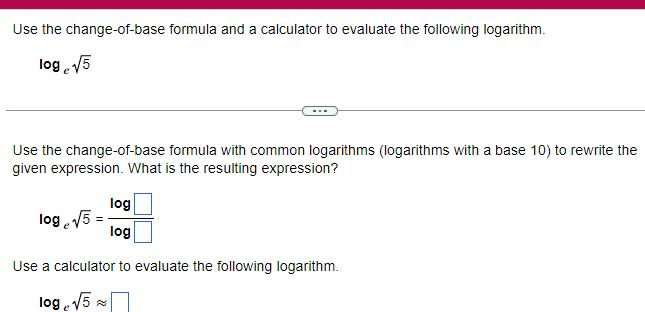Question:

# Use the change of base formula and a calculator to evaluate

Last updated: 11/21/2023Use the change of base formula and a calculator to evaluate the following logarithm log 5 Use the change of base formula with common logarithms logarithms with a base 10 to rewrite the given expression What is the resulting expression log log Use a calculator to evaluate the following logarithm log 5 log 5